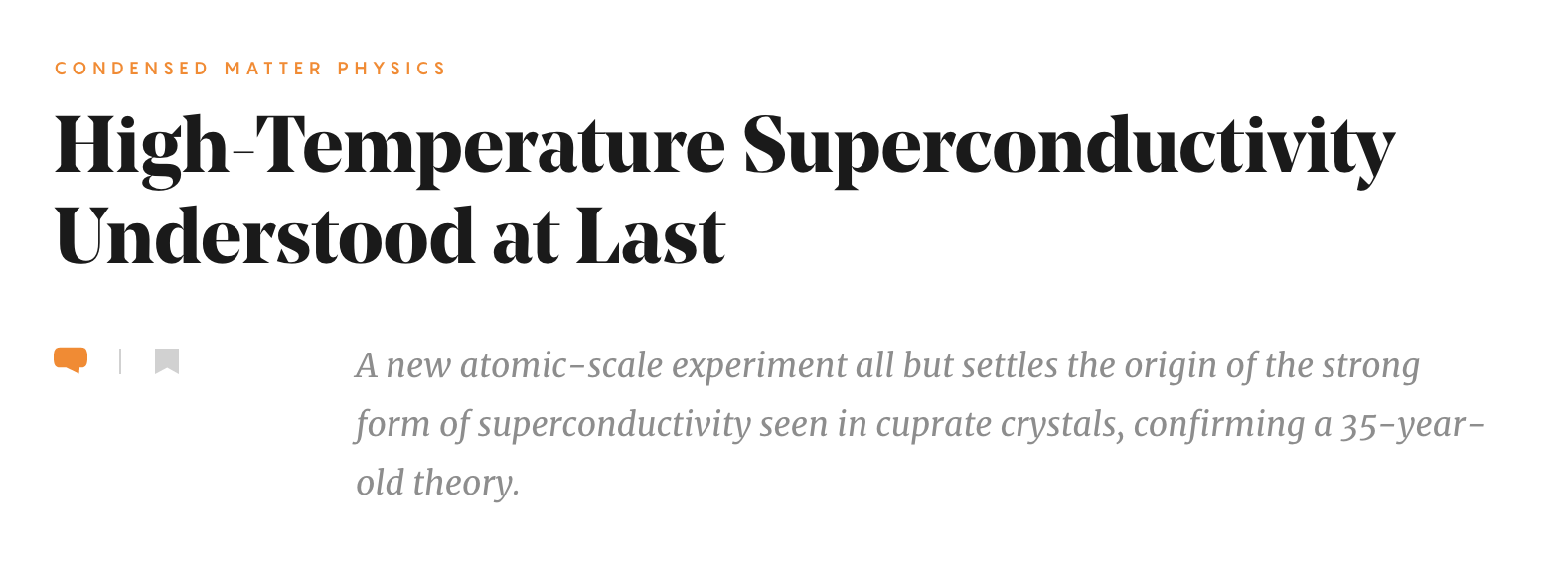# Lattice Models

• Defined on a discrete lattice
• Apply to deep periodic potentials: few states / site are enough
• Capture physics of strong correlations

## Tight Binding Models

• Noninteracting Hamiltonian with periodic potential $V(x+a)=V(x)$

$$H = \sum_{j=1}^N \left[-\frac{1}{2m}\partial_i^2 +V(x_i)\right] = \int \left[\frac{1}{2m}\partial_x\pdop\partial_x\pop + V(x)\pdop\pop\right] dx,$$

• Bloch’s theorem: eigenstates labelled by continuous index $k$ (crystal momentum) and discrete index $n$ (band index) $$\psi_{k,n}(x) = e^{ikx} \varphi_{k,n}(x),$$ $\varphi_{k,n}$ is periodic; $k$ lies in Brillouin zone $(-\pi/a,\pi/a]$

• The eigenvalues $E_n(k)$ give the energy bands.

• When lattice potential deep wavefunctions in lowest band(s) highly localized

• Introduce $\adop_j$, $\aop_j$ describing localized state $\varphi_j(x)$ at site $j$

• $\varphi_j(x)$ not eigenstates: coupling between sites described by tight binding Hamiltonian

$$H_t = -t \sum_{j} \left[\adop_j\aop_{j+1}+\adop_{j+1}\aop_j\right]$$

• Plays role of kinetic energy in our models (c.f. magnon in spin chain in Lecture 4

## Bose Hubbard Model

• Simplest interacting lattice model $$H = H_t + H_U = -t \sum_{\langle j\,k\rangle} \left[\adop_j\aop_{k}+\adop_{k}\aop_j\right] + \frac{U}{2}\sum_j N_j(N_j-1),$$ $N_j=\adop_j\aop_j$, sum over all nearest neighbours

• On-site interaction $N_j(N_j-1)=\adop_j\adop_j\aop_j\aop_j$

• First introduced to describe electrons in solids; boson version widely used in ultracold atomic physics

### The Mott State

• $U=0$ limit easy (no interactions); what about $U/t\to\infty$?

$$E(\mathbf{N}) = \frac{U}{2} \sum_j N_j(N_j-1).$$

• Ground state: fill $N_\text{sites}$ sites as uniformly as possible

• Easy if filling $\nu \equiv N_{\text{particles}}/N_\text{sites}$ is integer

• Notation: ceiling $\lceil \nu\rceil$ is smallest integer bigger than $\nu$, floor $\lfloor \nu\rfloor$ is largest integer smaller than $\mu$

• Number of sites with occupancy $\lceil \nu\rceil$ is $N_\text{sites}\left(\nu - \lfloor \nu\rfloor\right)$

• Ground state energy is piecewise linear in $\nu$ $$\frac{E_0}{N_\text{sites}} = \left(\nu - \lfloor \nu\rfloor\right)e(\lceil \nu\rceil) + \left(\lceil \nu\rceil - \nu\right)e(\lfloor \nu\rfloor),$$ $e(N) = \frac{U}{2}N(N-1)$

• Chemical potential $\mu = \frac{\partial E_0}{\partial N_\text{particles}}$ is piecewise constant

$$\mu = e(\lceil \nu\rceil) -e(\lfloor \nu\rfloor)=U\lfloor \nu\rfloor,$$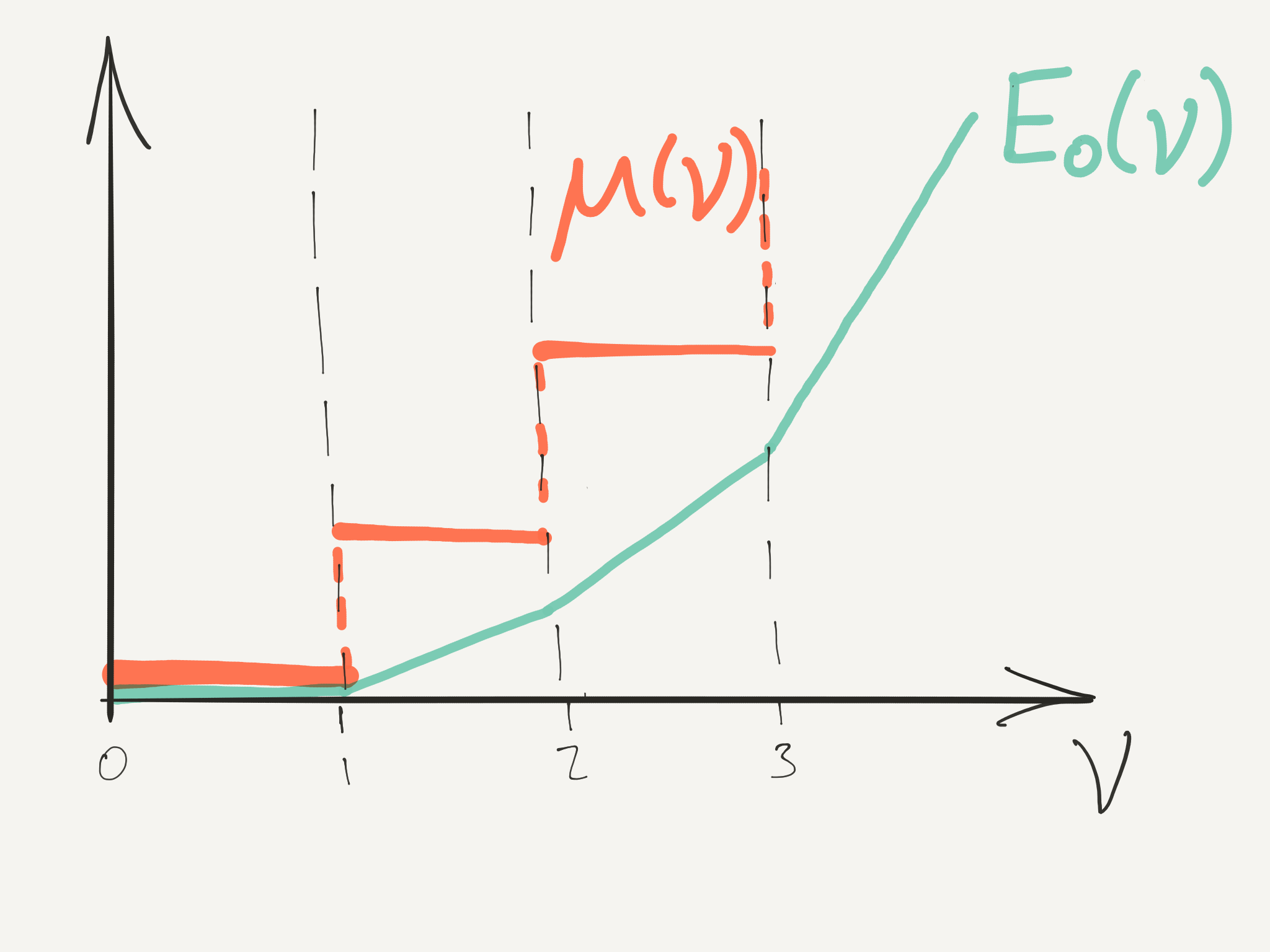• Think about varying $\mu$: values between $UN$ and $U(N-1)$ correspond to integer filling $\nu=N$

• States of integer filling are Mott states, after Nevill Mott

### Effect of hopping: Mott states

• Do Mott states survive when $t\neq 0$? Treat $t$ as perturbation

• Unperturbed eigenstates of have fixed occupation $N_j$ on site $j$

$$\ket{\mathbf{N}} = \bigotimes_j \ket{N_j}_j.$$

• Applying $H_t$ gives a superposition of states, each with one particle moved to an adjacent site.

How is a Mott state (all sites with the same occupation) affected by $H_t$ in first order perturbation theory?

### Effect of hopping: away from Mott state

• Mott state of filling $\nu=N$ + 1 extra particle

• Place particle on one of $N_\text{sites}$ sites: degnerate ground state when $t=0$

• $H_t$ mixes these states: degenerate perturbation theory (other states separated by energies of order $U$)

• Ground state multiplet

$$\ket{i,+}\equiv \frac{\adop_i}{\sqrt{N+1}}\bigotimes_{j} \ket{N}_j$$

• Only states $\ket{i\pm 1,+}$ are coupled by $H_t$, with matrix elements

$$\braket{j|H_t|k} = -t(N+1)$$

• Within degnerate multiplet, $H_t$ corresponds to tight binding model

$$H_t\rvert_{+} = -t(N+1) \sum_{\langle j,k\rangle} \left[\ket{j,+}\bra{k,+}+\text{h.c.}\right]$$

$$H_t\rvert_{+} = -t(N+1) \sum_{\langle j,k\rangle} \left[\ket{j,+}\bra{k,+}+\text{h.c.}\right].$$

• Only many body effect is factor of $N$ due to Bose statistics

• Splitting of degenerate states given by dispersion ($d$ dimensions)

$$\omega_+(\boldsymbol{\eta}) = -2t(N+1)\sum_{n=1}^d\cos\eta_n$$

• Alternatively remove a particle from Mott state

$$\ket{i,-}\equiv \frac{\aop_i}{\sqrt{N}}\bigotimes_{j} \ket{N}_j.$$

• Within these states, $H_t$ takes form

$$H_t\rvert_{-}=-tN \sum_{\langle j,k\rangle} \left[\ket{j,-}\bra{k,-}+\text{h.c.}\right]$$

$$\omega_-(\boldsymbol{\eta}) = -2tN\sum_{n=1}^d\cos\eta_n.$$

### Changes to phase diagram

• Introduce the grand canonical Hamiltonian

$$\ch_\mu = H - \mu N_\text{particles},$$

• At $t=0$ the energies of Mott states with filling $\nu=N$ are $$\frac{\ce^{(N)}_\mu}{N_\text{sites}} = \frac{U}{2}N(N-1)-\mu N.$$ $\ce^{(N)}_\mu$ and $\ce^{(N+1)}_\mu$ degenerate when $\mu=UN$ for $t=0$
• Compare with ground state with one extra particle on top of $N$ Mott state

• Particle at bottom of tight binding band has energy $-2td(N+1)$

• Together with extra energy of interaction we have overall

$$\ce^{(N)}_\mu + UN - \mu -2dt(N+1).$$

• For $t>\frac{UN-\mu}{2d(N+1)}$ state with an extra particle actually has a lower energy: Mott state not the ground state
• Energy of ground state with one ‘hole’ in $N+1$ Mott state is

$$\ce^{(N+1)}_\mu - UN + \mu -2dt(N+1).$$

• Introducing a hole is thus favoured for $t>\frac{\mu-UN}{2d(N+1)}$.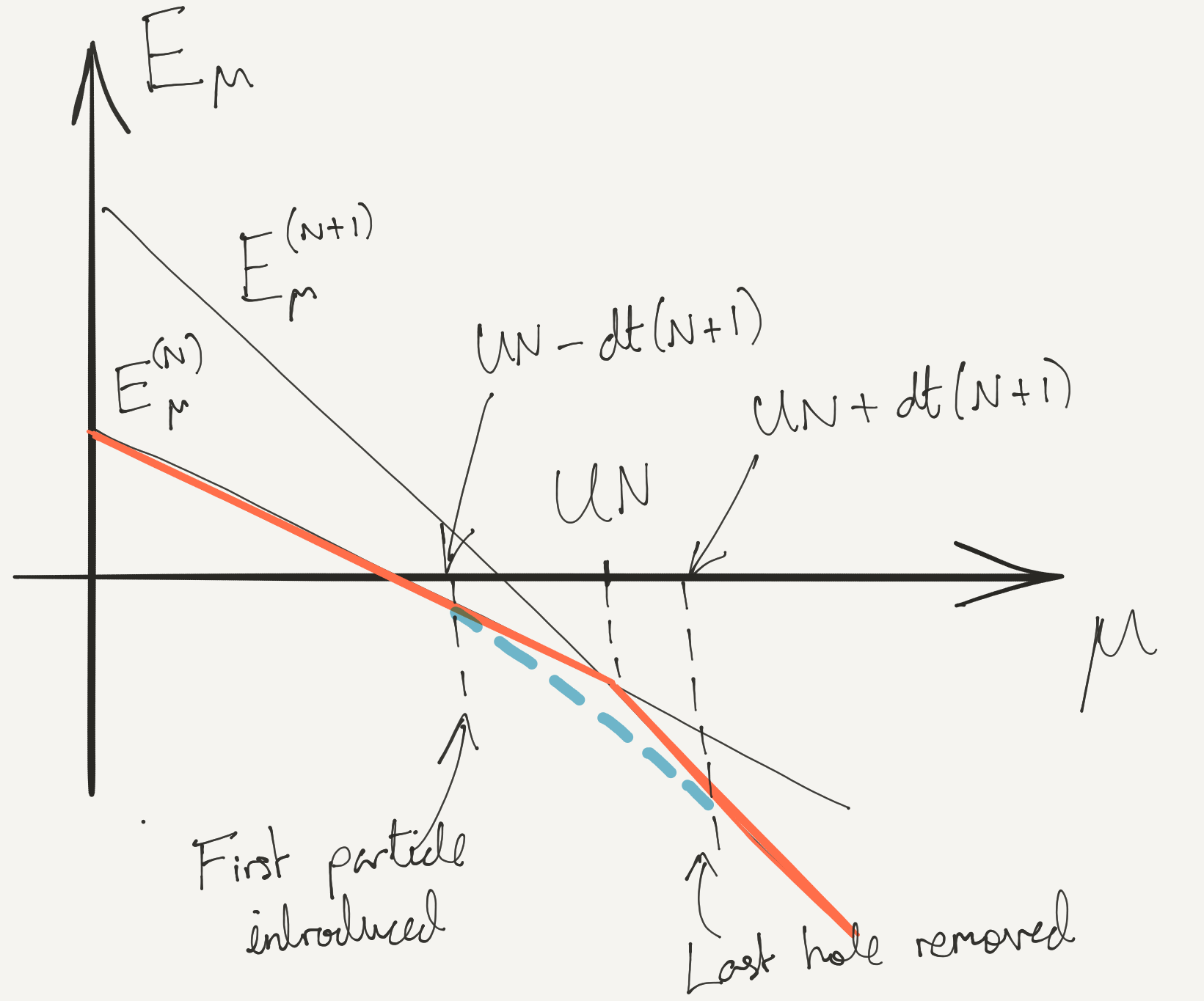• Our analysis applies only at small $t/U$

• If $t/U\to\infty$ we have a Bose condensate: all the particles can sit in the $\eta=0$ Bloch state

• When interactions are finite but small, we will see in the next lecture that the result is a superfluid.

• The boundaries that we have have found can be connected (Problem Set 2 for a variational approach)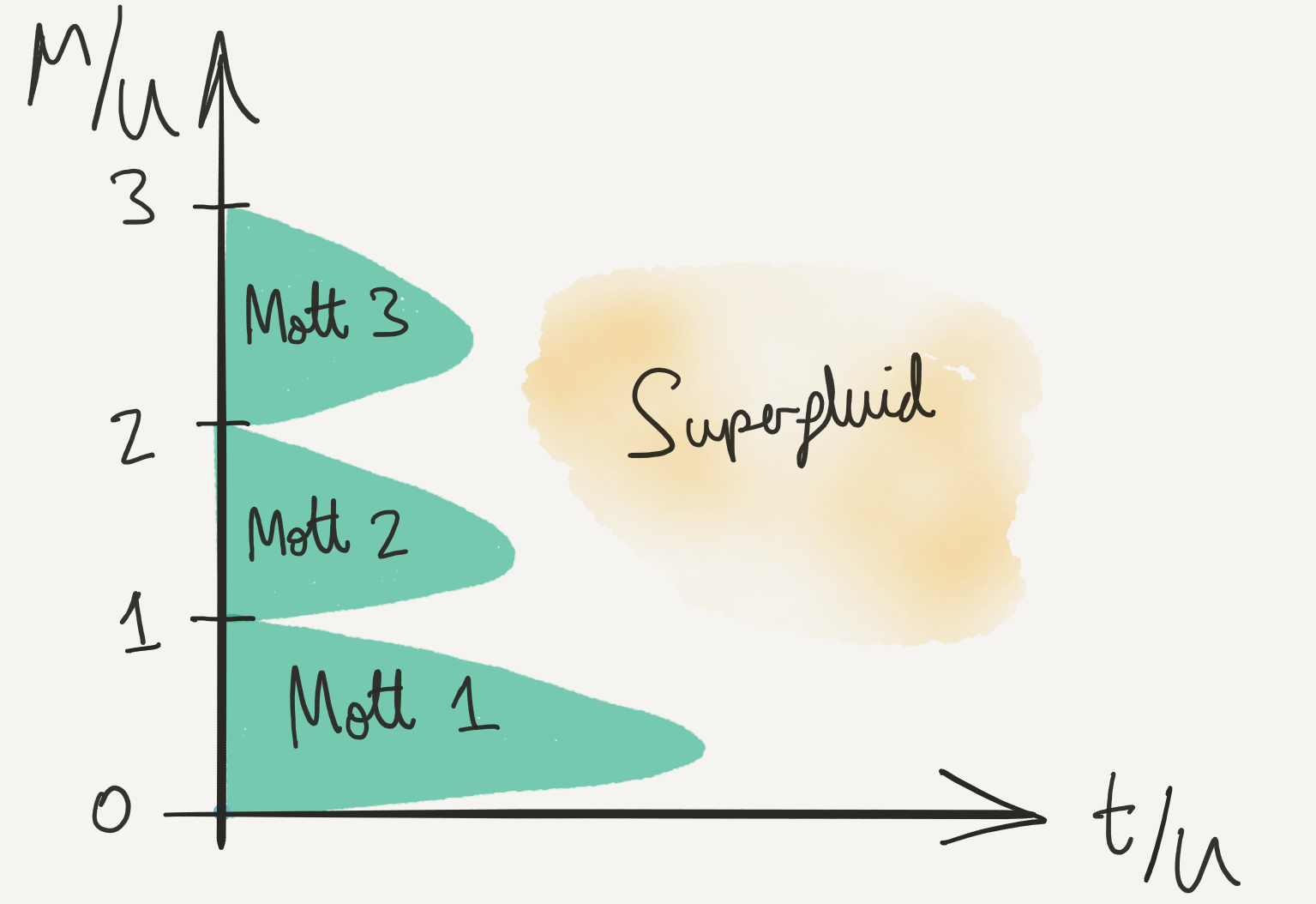• Note diminishing size of Mott lobes: consequence of enhanced hopping in the effective tight binding models• Mott wedding cake (Source: Cheng Chin, University of Chicago)

## Fermi Hubbard Model

• Have to introduce spin to get something interesting (why?)

$$H=-t \sum_{\substack{\langle j,k\rangle\ s=\uparrow,\downarrow}} \left[\adop_{j,s}\aop_{k,s}+\adop_{k,s}\aop_{j,s}\right] + U\sum_j N_\uparrow N_\downarrow,$$

• Application cuprates: high temperature superconductors

• 2D model describes strong correlations in the CuO$_2$ layers

• Much harder to understand than Bose case…

### $U/t\to \infty$

• Three Mott staes to 0, 1, or 2 particles per site

• 2 particles on site make a spin singlet $\frac{1}{\sqrt{2}}(\ket{\uparrow}\ket{\downarrow}-\ket{\downarrow}\ket{\uparrow})$

• At half filling (1 particle / site) can have $\ket{\uparrow/\downarrow}$.

• $\nu=1$ Mott state is massively degenerate, with a ground state multiplet consisting of $2^{N_\text{sites}}$ possible spin configurations

• What happens when $U/t$ large but finite?

### Two Sites, Two Fermions

• 6 states altogether in the Hilbert space

\begin{align*} \adop_{1,\uparrow}\adop_{1,\downarrow} \ket{\text{VAC}},\quad\adop_{2,\uparrow}\adop_{2,\downarrow} \ket{\text{VAC}}\\ \adop_{1,s}\adop_{2,s'} \ket{\text{VAC}},\quad s,s'=\uparrow,\downarrow. \end{align*}

• Top two states have energy $U$ when $t=0$; rest have energy 0.

[Try this before continuing!] How does the degeneracy of these states get lifted at finite $t$? Try writing down the Hamiltonian restricted to these states.

### Effective Hamiltonian

• Find effective Hamiltonian $H_\text{eff}$ that acts only on the half filled Mott states to describe splitting when $t/U\neq 0$ is finite

• Solving the $H_\text{eff}$ comes later!

• Need second order degenerate perturbation theory because $H_t$ has zero matrix elements in degenerate subspace

• Hamiltonian has block structure, with off-diagonal block $V\propto t$

$$H = \begin{pmatrix} H_{\text{Mott}} & V^{} \\ V^\dagger & H_\text{Not} \\ \end{pmatrix}$$

• Notation: $P_\text{Mott}$ is projection operator on to the $2^{N_\text{sites}}$ Mott states \begin{align*} H_\text{Mott}= P_\text{Mott} H P_\text{Mott},\quad H_\text{Not}= P_\text{Not}H P_\text{Not}\\ V^{} = P_\text{Mott} H P_\text{Not},\qquad V^\dagger = P_\text{Not} H P_\text{Mott}. \end{align*} $P_\text{Not}\equiv 1-P_\text{Mott}$
• For Hubbard model \begin{align*} H_\text{Mott}= P_\text{Mott} H_U P_\text{Mott},\quad H_\text{Not}= P_\text{Not}H_U P_\text{Not}\\ V^{} = P_\text{Mott} H_t P_\text{Not},\qquad V^\dagger = P_\text{Not} H_t P_\text{Mott}. \end{align*} $H_{\text{Mott}}=0$ ($\nu=1$ states have no interaction energy)

• Write eigenvalue equation in block form

$$\begin{pmatrix} 0 & V^{} \\ V^\dagger & H_\text{Not} \\ \end{pmatrix} \begin{pmatrix} \ket{\Psi}\\ \ket{\Phi} \end{pmatrix} = E \begin{pmatrix} \ket{\Psi}\\ \ket{\Phi} \end{pmatrix}.$$

• Eliminate $\ket{\Phi}$

$$-V^{}\left(H_\text{Not}-E\right)^{-1}V^\dagger\ket{\Psi} = E\ket{\Psi}.$$

$$-V^{}\left(H_\text{Not}-E\right)^{-1}V^\dagger\ket{\Psi} = E\ket{\Psi}.$$

• Not an eigenvalue equation because of $E$ on LHS

• But if $E\ll$ eigenvalues of $H_\text{Not}=O(U)$

$$H_\text{eff} =-V^{} H^{-1}_\text{Not}V^\dagger.$$

### Form of $H_\text{eff}$

$$H_\text{eff} =-V^{} H^{-1}_\text{Not}V^\dagger.$$

1. $V^\dagger$ creates states with adjacent hole and doublon (doubly occupied site)

2. $H_\text{Not}$ acting on these states is just $U$

3. $V$ has to remove the hole and doublon

$$H_\text{eff} = -\frac{V^{}V^\dagger}{U} = -\frac{t^2}{U} \sum_{\langle j,k\rangle s,s’} \left[\adop_{j,s}\aop_{k,s} \adop_{k,s’}\aop_{j,s’}+j\leftrightarrow k\right]$$

$$H_\text{eff} = -\frac{V^{}V^\dagger}{U} = -\frac{t^2}{U} \sum_{\langle j,k\rangle s,s’} \left[\adop_{j,s}\aop_{k,s} \adop_{k,s’}\aop_{j,s’}+j\leftrightarrow k\right]$$

• Re-write by first reordering the operators (anticommutation!)

$$\adop_{j,s}\aop_{k,s} \adop_{k,s’}\aop_{j,s’} = -\adop_{j,s}\aop_{j,s’}\adop_{k,s’}\aop_{k,s} + \delta_{s^{}s’}\adop_{j,s}\aop_{j,s’}$$

• Then use identity

$$\delta_{ab}\delta_{cd} = \frac{1}{2}\left[\boldsymbol{\sigma}_{a d}\cdot \boldsymbol{\sigma}_{c b} + \delta_{ad}\delta_{cb}\right]$$

• In $d$ dimensions ($d=1$, chain; $d=2$ square lattice; $d=3$ cubic lattice) $$H_\text{eff} = -\frac{dN_\text{sites}t^2}{2U}+J\sum_{\langle j\,k\rangle} \mathbf{s}_j\cdot \mathbf{s}_k$$ with $J=\frac{2t^2}{U}$ and

$$\mathbf{s}_j=\frac{1}{2}\sum_{s,s'}\adop_{j,s}\boldsymbol{\sigma}_{s^{}s'}\aop_{j,s'}.$$

• $H_\text{eff}$ is spin-1/2 antiferromagnetic Heisenberg model!

### Doping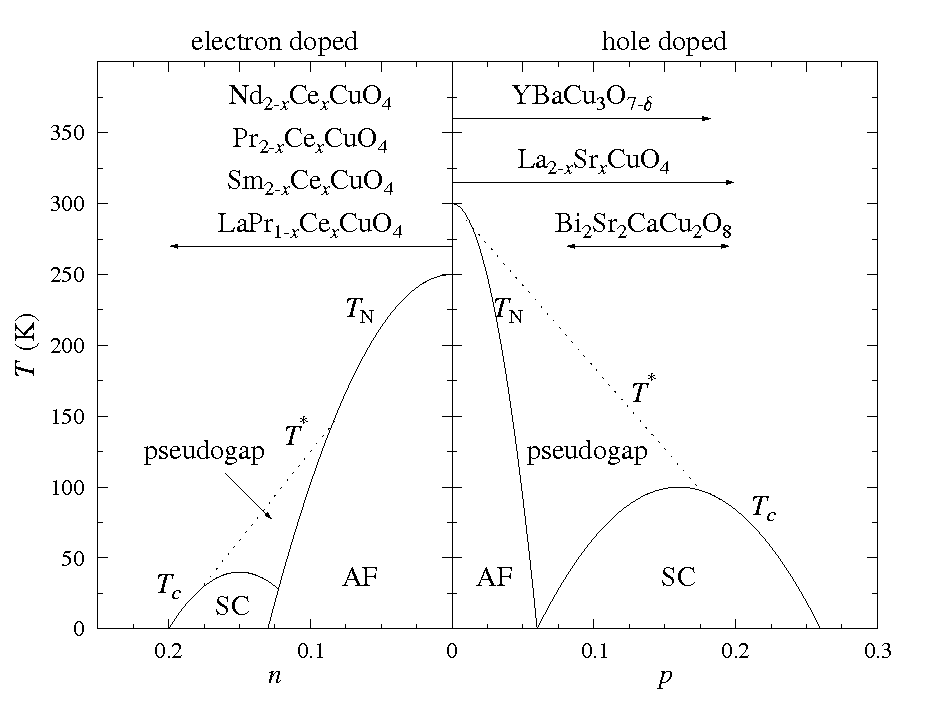• At half filling, cuprates are antiferromangetic Mott insulators

• Superconductivity emerges when materials doped, introducing electrons or holes into CuO$_2$ planes

• Superconductivity appears where antiferromagnetism dies. Why?

### t-J model

• Effective Hamiltonian that describes doped Mott insulator

$$H_\text{eff} = -t \sum_{\substack{\langle j,k\rangle\ s=\uparrow,\downarrow}} \left[\adop_{j,s}\aop_{k,s}+\adop_{k,s}\aop_{j,s}\right] + J\sum_{<j,k>}\left[\mathbf{s}_j\cdot \mathbf{s}_k - \frac{N_j N_k}{4}\right]$$

• Hopping term allows holes or doubly occupied sites to move

• Heisenberg exchange term only acts between sites with one particle. $\mathbf{s}_j$ vanish on empty or doubly occupied sites